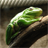# Need help returning total sum of table field

803
4
04-04-2017 10:53 AMNew Contributor

Hi I'm having trouble returning the total sum of my value field to my updateFC for each row within the update feature class. Any pointers would be appreciated.

``````import arcpy
import numpy as np
arcpy.env.workspace = 'C:\Users\michellep\Documents\project.gdb'

print "Arcpy & Numpy Successfully Imported"
print "Start Processing"
start = time.time()

sourceFC = 'Test_Group'
sourceFields = ['ID_Data', 'KV', 'AV', 'Total']
pc = r'C:\Users\michellep\Documents\Data\Table.dbf'
PCFields = ['AV', 'KV', 'WV', 'B', 'kW', 'Value']

#create dict of test group

valueDict = {r:(r[1:]) for r in arcpy.da.SearchCursor(sourceFC, sourceFields)}

#point to update FC test_group_1
updateFC = 'Test_Group_1'
updateFields = ['ID_Data', 'KV', 'AV', 'Total']

updateCursor = arcpy.da.UpdateCursor(updateFC, updateFields)
updatePC = arcpy.da.UpdateCursor(pc, PCFields)

for UpdateRow in updateCursor:
keyValue = UpdateRow
if keyValue in valueDict:
for row in updatePC:
row = valueDict[keyValue]
row = valueDict[keyValue]
row = row/ row * (row/ row)
row = row * row
updatePC.updateRow(row)
arr = arcpy.da.TabletoNumPyArray(pc, "Value")
arr.dtype = np.dtype(float)
UpdateRow = np.sum(arr)
del row
updateCursor.updateRow(UpdateRow)
del UpdateRow

end = time.time()
processTime = end - start
print "Processing Completed - {:.1f} seconds".format(processTime)‍‍‍‍‍‍‍‍‍‍‍‍‍‍‍‍‍‍‍‍‍‍‍‍‍‍‍‍‍‍‍‍‍‍‍‍‍‍‍‍‍‍‍‍‍‍‍‍‍‍‍‍‍‍‍‍‍‍‍‍‍‍‍‍‍‍‍‍‍‍‍‍‍‍‍‍‍‍‍‍‍‍‍‍‍‍‍‍``````
4 RepliesFrequent ContributorFrequent Contributor

Thanks for fixing the basic formatting, but the spacing is still off.  If you could fix the indentation also that would be good.

I think the issue is you set all the values for your fields in PC Fields, but do not update the row prior to making your numpy array to sum up the values of the VALUE field.  I would guess you would need to update the row first, then create the numpy array for the values.New Contributor

Hi Ian

Thanks for the reply.  I fixed the indentation, I also have updated the code somewhat.  As you suggested I updated the row first then created the numpy array but now the script is only returning one value to the updateRow field when i need a returned value for updateRow for each row in the updateFC.Frequent Contributor

I'm guessing you are basing this off of Richard Fairhurst's Blog Post: https://community.esri.com/blogs/richard_fairhurst/2014/11/08/turbo-charging-data-manipulation-with-...

I think you have several significant issues here.  First you are trying to update values for PC based on I'm assuming a related field in source FC.  You never establish a related field and instead are rewriting the values every time for 'AV', 'KV', 'WV', and Value for each record in Update FC.  Second you create a numpy array each and every time through and it would change each time.  Third you are deleting your row and UpdateRow unnecessarily early in your script.  Fourth, you are nesting cursors which is never ever a good idea, update the values in your first feature, then create a numpy array so sum the new values in the Value field, then use an new cursor to update the new values into the Update FC.  I'm guessing the Total field should have the exact same value for every record in your Update FC since you are trying to sum up the entirety of the Value Field to place into it.

I'm operating on alot of assumptions with what I posted above.  I think the best thing you could do is give more information about what exactly you are trying to do, screenshots of your tables/fields would be useful.  Right now you are asking us for help fixing your code, without ever really saying what its supposed to be doing.